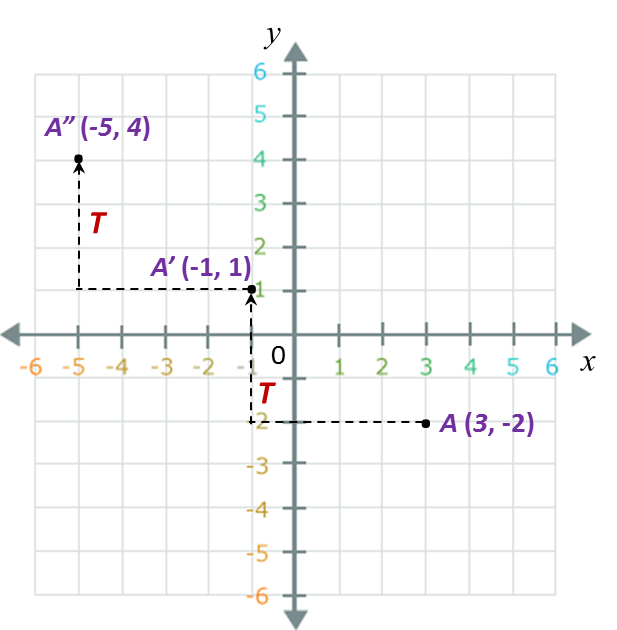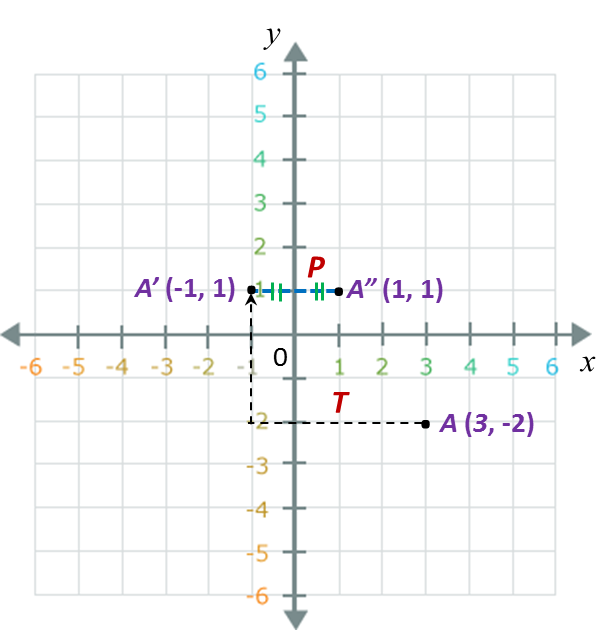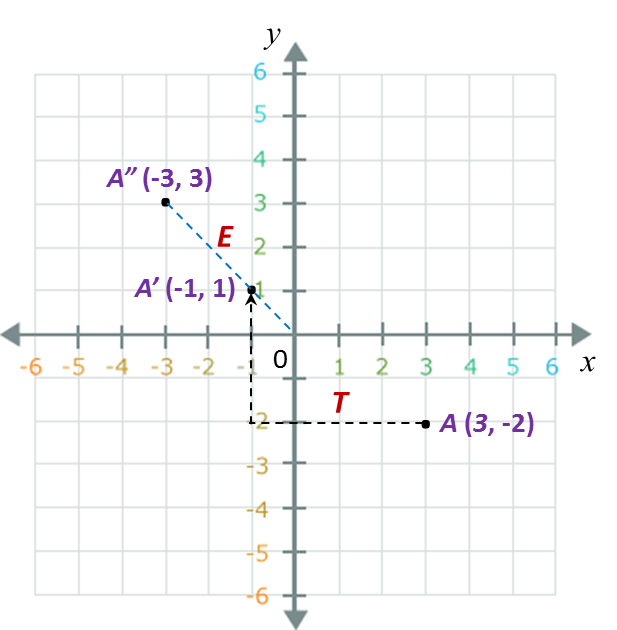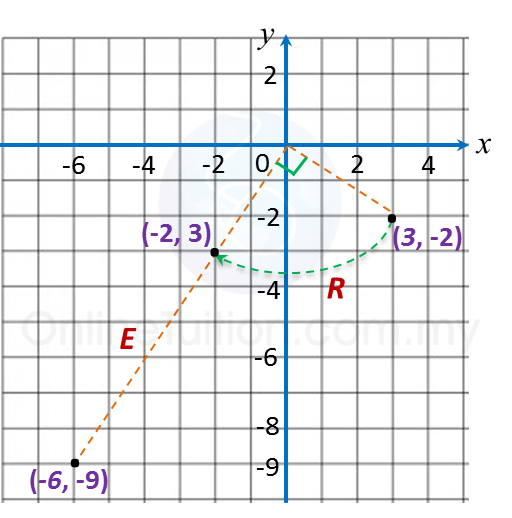# 3.1c Stating the Coordinates of the Image of a Point under a Combined Transformation

3.1c Stating the Coordinates of the Image of a Point under a Combined Transformation

1. The coordinates of the image of a point, K, under the combined transformation AB can be determined by the following steps.

Step 1:
Determine the coordinates of K’, the image of K, under the first transformation, B.

Step 2:
Determine the coordinates of K”, the image of K’, under the second transformation, A. K” is the image of K, under the combined transformation, AB.

Example:
T, P, R and E are four transformations that are defined as follows:
$T=Translation\text{}\left(\begin{array}{l}-4\\ \text{}3\end{array}\right)$
= Reflection in the y–axis.
= Clockwise rotation 90o about the origin
= Enlargement with scale factor of 3 and the origin as centre.
Find the coordinates of the image of the point A (3, –2) under each of the following combined transformation.
(a) TT (b) PT (c) ET (d) ER  (e) EP

Solution:
(a)A(3, –2) → TA’(–1, 1) → TA”(–5, 4).

(b)A(3, –2) → TA’(–1, 1) → PA”(1, 1).

(c)A(3, –2) → TA’(–1, 1) → EA”( –3, 3).

(d)A(3, –2) → RA’(–2, 3) → EA”( –6, –9).

(e)A(3, –2) → PA’(–3, –2) → EA”( –9, –6).